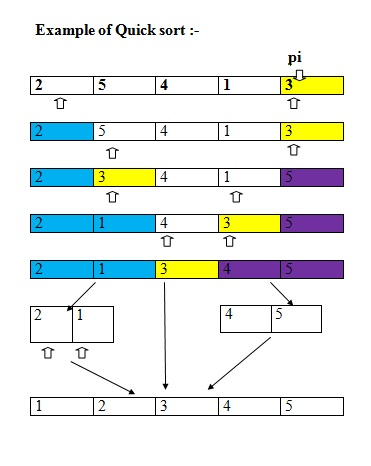# Quick Sort in C++ with Algorithm, Example

Learn: Quick Sort in C++ with Example, Algorithm. Quick sort is a sorting technique of Data Structure, here we will learn quick sort implementation using C++.
Submitted by Amit Shukla, on June 09, 2017

It was invented by Sir Tony Hoare in 1959.

In quick sort we split the array into two parts and all the elements of one part is less than or equal to elements of other part for all possible indexes for both parts and if we sort these lines repeatedly then the entire array will be sorted. These are known as Divide and conquer approach.

## Description of quick sort

Pick a random pivot element pi , from, a partition a into the set of elements less than pi , the set of elements equal to pi , and the set of elements greater than pi and finally, recursively sort the first and third sets in this partition.

Algorithm:

```quickSort(array<T>& a)
{
quickSort(a, 0, a.length);
quickSort(array<T> & a, int i, int n)
{
if (n <= 1)
return;
T pi = a[i + rand() % n];
int p = i - 1, j = i, q = i + n;
while (j < q) {
int comp = compare(a[j], pi);
if (comp < 0) {
a.swap(j++, ++p); // move to beginning of array
}
else if (comp > 0) {
a.swap(j, --q); // move to end of array
}
else {
j++; // keep in the middle
}
}
}
}
// a[i..p]<pi, a[p+1..q-1]=pi, a[q..i+n-1]>pi
quickSort(a, i, p - i + 1);
quickSort(a, q, n - (q - i));
```

Consider the Quick sort working flow:### C++ program for Quick Sort

```#include <iostream>
using namespace std;

void quicksort(int, int, int);
int partition(int, int, int);

int partition(int* a, int s, int e)
{
int piviot = a[e];
int pind = s;
int i, t;

for (i = s; i < e; i++) {
if (a[i] <= piviot) {
t = a[i];
a[i] = a[pind];
a[pind] = t;
pind++;
}
}

t = a[e];
a[e] = a[pind];
a[pind] = t;

return pind;
}

void quicksort(int* a, int s, int e)
{
if (s < e) {
int pind = partition(a, s, e);
quicksort(a, s, pind - 1);
quicksort(a, pind + 1, e);
}
}

int main()
{
int n;

cout << "Enter number of elements : " ;
cin >> n;

int a[n];

cout << "Enter the array : ";
for (int i = 0; i < n; i++) {
cin >> a[i];
}

quicksort(a, 0, n - 1);

cout << "Sorted array is : ";
for (int i = 0; i < n; i++) {
cout << a[i] << " ";
}

return 0;
}
```

Output

```RUN 1:
Enter number of elements : 5
Enter the array : 10 20 5 15 10
Sorted array is : 5 10 10 15 20

RUN 2:
Enter number of elements : 10
Enter the array : 40 30 20 50 60 70 80 90 10 11
Sorted array is : 10 11 20 30 40 50 60 70 80 90
```